# Factorial Number Program in C#

## Factorial Number Program in C# with Examples

In this article, I am going to discuss the Factorial Number Program in C# with Examples. Please read our previous article where we discussed the Armstrong Number Program in C# with examples. In many interviews, the interviewer asked this question in many different ways. As part of this article, we are going to discuss the following pointers.

1. What is factorial of a number?
2. Factorial of a number using for loop, while loop and do-while loop in C#.
3. Factorial of a number using the recursive function in C#.
##### What is Factorial of a number?

The Factorial of a number (let say n) is nothing but the product of all positive descending integers of that number. Factorial of n is denoted by n!. Please have a look at the following image which shows how to calculate the factorials of a number.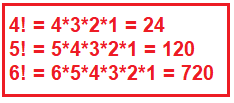##### Factorial Number Program in C# using for loop:

In the following example, we take the number from the console and then calculate the factorial of that number using for loop.

```using System;
namespace LogicalPrograms
{
class Program
{
static void Main(string[] args)
{
Console.Write("Enter a Number : ");

int factorial = 1;
for (int i = 1; i <= number; i++)
{
factorial = factorial * i;
}
Console.Write(\$"Factorial of {number}  is: {factorial}");

}
}
}
```
###### Output: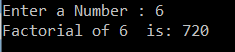##### Factorials of a number using while loop in C#:

In the following example, we use while loop to calculate the factorial of a number.

```using System;
namespace LogicalPrograms
{
class Program
{
static void Main(string[] args)
{
Console.Write("Enter a Number : ");

long factorial = 1;
while (number != 1)
{
factorial = factorial * number;
number = number - 1;
}

Console.Write(\$"Factorial is: {factorial}");
}
}
}
```
###### Output: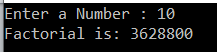##### Factorial of a number using Recursive Function in C#:

In the following program, we use a recursive function to calculate the factorial of a given number.

```using System;
namespace LogicalPrograms
{
class Program
{
static void Main(string[] args)
{
Console.Write("Enter a Number : ");

long factorial = RecursiveFactorial(number);
Console.Write(\$"Factorial of {number} is: {factorial}");

}

static long RecursiveFactorial(int number)
{
if (number == 1)
{
return 1;
}
else
{
return number * RecursiveFactorial(number - 1);
}
}
}
}
```
###### Output: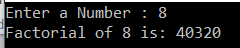##### Factorial of a number using the do-while loop in C#:

In the below program, we use the do-while loop to calculate the factorial of a given number. The number here we are taking from the console.

```using System;
namespace LogicalPrograms
{
class Program
{
static void Main(string[] args)
{
Console.Write("Enter a Number : ");
long factorial =  1;

do
{
factorial = factorial * number;
number--;
} while (number > 0);

Console.Write(\$"The Factorial is: {factorial}");
}
}
}
```
###### Output: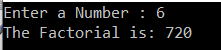In the next article, I am going to discuss the Sum Of Digits Program in C# with some examples. Here, in this article, I try to explain the different ways to implement the Factorial Number Program in C# with examples.

### 1 thought on “Factorial Number Program in C#”

1.the great for loop

How about I don’t need input by the user I want to pop up the factorial 1-20 ?